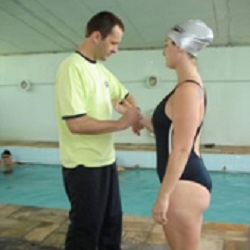## CEC CourseImprove the quality of your cardiorespiratory workouts for your clients! Learn how to have your clients working in their target heart rate zones to maximize weight loss and effectively improve function. This course provides evidence based methodology and practice for the often confusing guidelines for aquatic heart rate deductions. Generally accepted equations and methods are provided with easy-to-follow explanations and practice equations. After completing this course you will be able to determine and apply aquatic target heart rates for safe and effective exercise intensity.

## Course Information

Authors:Aquatic Exercise Association

Prerequisites: None

• Online Interactive Course
• Online Quiz (18 multiple choice questions. Minimum passing score 75%)
After completing this course you will be able to:
1. Know definitions for heart rate terms.
2. Learn how to calculate a maximum heart rate using the Standard HR Equation and the Gellish Equation.
3. Learn how to calculate a target heart rate intensity using the Percentage of Maximum Heart Rate formula and the Karvonen formula.
4. Understand the use of Rate of Perceived Exertion in measuring exercise intensity.
5. Know the theory and causes for aquatic heart rate responses.
6. Learn the evolution of aquatic heart rate deductions.
7. Learn the Kruel Protocol for Individualized Heart Rate Deductions.
8. Learn, understand, and practice 2 methods for determining aquatic target heart rates.
9. Understand how to measure and interpret aquatic heart rates.
10. Practice equations for maximum heart rate and target heart rate for both land and water.